Download Presentation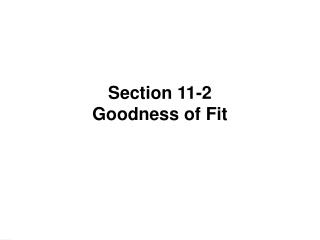Section 11-2 Goodness of Fit

# Section 11-2 Goodness of Fit - PowerPoint PPT Presentation

Section 11-2 Goodness of Fit. Key Concept. Sample data consist of observed frequency counts arranged in a single row (called a one-way frequency table). We will test the claim that the observed frequency counts agree with some claimed distribution .I am the owner, or an agent authorized to act on behalf of the owner, of the copyrighted work described.
Download Presentation## Section 11-2 Goodness of Fit

An Image/Link below is provided (as is) to download presentation

Download Policy: Content on the Website is provided to you AS IS for your information and personal use and may not be sold / licensed / shared on other websites without getting consent from its author.While downloading, if for some reason you are not able to download a presentation, the publisher may have deleted the file from their server.

- - - - - - - - - - - - - - - - - - - - - - - - - - E N D - - - - - - - - - - - - - - - - - - - - - - - - - -
Presentation Transcript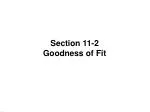Section 11-2

Goodness of Fit

.

.Key Concept

Sample data consist of observed frequency counts arranged in a single row

(called a one-way frequency table).

We will test the claim that the observed frequency counts agree with some claimed distribution.

In other words, there is a good fit of the observed data with the claimed distribution.

.

.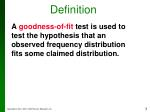Definition

A goodness-of-fit test is used to test the hypothesis that an observed frequency distribution fits some claimed distribution.

.

.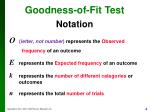O (letter, not number) represents the Observed

frequency of an outcome

E represents the Expected frequency of an outcome

krepresents the number of different categories or outcomes

nrepresents the total number of trials

Goodness-of-FitTest

Notation

.

.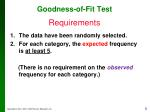Goodness-of-Fit Test

The data have been randomly selected.

For each category, the expected frequency is at least 5.

(There is no requirement on the observedfrequency for each category.)

Requirements

.

.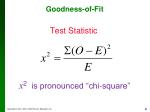Goodness-of-Fit

Test Statistic

x2 is pronounced “chi-square”

.

.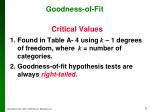Goodness-of-Fit

Critical Values

1. Found in Table A- 4 using k – 1 degrees of freedom, where k = number of categories.

2. Goodness-of-fit hypothesis tests are always right-tailed.

.

.Goodness-of-Fit

P-Values

P-values are typically provided by computer software, or a range of P-values can be found from Table A-4.

.

.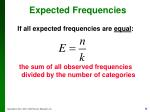Expected Frequencies

If all expected frequencies are equal:

the sum of all observed frequencies divided by the number of categories

.

.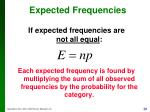If expected frequencies arenot all equal:

Each expected frequency is found by multiplying the sum of all observed frequencies by the probability for the category.

Expected Frequencies

.

.A largedisagreement between observed and expected values will lead to a large value of and a small P-value.

A significantlylarge value of will cause a rejection of the null hypothesis of no difference between the observed and the expected.

Goodness-of-Fit Test
• A close agreement between observed and expected values will lead to a small value of and a large P-value.

.

.Goodness-of-Fit Test

“If the P is low, the null must go.”

(If the P-value is small, reject the null hypothesis that the distribution is as claimed.)

.

.Example:

Data Set 1 in Appendix B includes weights from 40 randomly selected adult males and 40 randomly selected adult females. Those weights were obtained as part of the National Health Examination Survey. When obtaining weights of subjects, it is extremely important to actually weigh individuals instead of asking them to report their weights. By analyzing the last digits of weights, researchers can verify that weights were obtained through actual measurements instead of being reported.

.

.Example:

When people report weights, they typically round to a whole number, so reported weights tend to have many last digits consisting of 0. In contrast, if people are actually weighed with a scale having precision to the nearest 0.1 pound, the weights tend to have last digits that are uniformly distributed, with 0, 1, 2, … , 9 all occurring with roughly the same frequencies. Table 11-2 shows the frequency distribution of the last digits from 80 weights listed in Data Set 1 in Appendix B.

.

.Example:

(For example, the weight of 201.5 lb has a last digit of 5, and this is one of the data values included in Table 11-2.)

Test the claim that the sample is from a population of weights in which the last digits do not occur with the same frequency. Based on the results, what can we conclude about the procedure used to obtain the weights?

.

.Example:

Requirements are satisfied: randomly selected subjects, frequency counts, expected frequency is

Step 1: at least one of the probabilities

, is different from the others

Step 2: at least one of the probabilities are the same:

Step 3: null hypothesis contains equality

: At least one probability is different

.

.Example:

Step 4: no significance specified, use

Step 5: testing whether a uniform distribution so use goodness-of-fit test:

Step 6: see the next slide for the computation of the test statistic. The test statistic

, using and degrees of freedom, the critical value is

.

.Example:

Step 7:

Because the test statistic does not fall in the critical region, there is not sufficient evidence to reject the null hypothesis.

.

.Example:

Step 8: There is not sufficient evidence to support the claim that the last digits do not occur with the same relative frequency.

This goodness-of-fit test suggests that the last digits provide a reasonably good fit with the claimed distribution of equally likely frequencies. Instead of asking the subjects how much they weigh, it appears that their weights were actually measured as they should have been.

.

.Goodness-of-fit by TI-83/84

• Press STAT and select EDIT
• Enter Observed frequencies into the list L1
• Enter Expected frequencies into the list L2
• then the procedure differs for TI-83 and TI-84:
• In TI-84: Press STAT, select TESTS
• scroll down to c2GOF-Test , press ENTER
• Type in: Observed: L1
• Expected: L2
• df: number of degrees of freedom
• Press on Calculate
• readthe test statistic c2=...
• and the P-value p=…Goodness-of-fit by TI-83/84

• In TI-83: Clear screen and type
• (L1- L2 )2 ÷L2 → L3
• (the key STOproduces the arrow → )
• Then press 2nd and STAT, select MATH
• scroll down to sum( press ENTER
• at prompt sum( type L3then) and press ENTER
• This gives you the c2test statistic
• For the P-value use the function c2 CDF
• fromDISTR menu and at prompt c2 CDF(
• type test_statistic,9999,df)